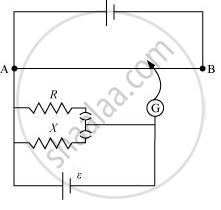Share

# Figure 3.34 Shows a Potentiometer Circuit for Comparison of Two Resistances. the Balance Point with a Standard Resistor R = 10.0 Ωis Found to Be 58.3 cm, While that with the Unknown Resistance X Is 68.5cm. - CBSE (Science) Class 12 - Physics

#### Question

Figure 3.34 shows a potentiometer circuit for comparison of two resistances. The balance point with a standard resistor R = 10.0 Ω is found to be 58.3 cm, while that with the unknown resistance is 68.5 cm. Determine the value of X. What might you do if you failed to find a balance point with the given cell of emf ε?#### Solution

Resistance of the standard resistor, R = 10.0 Ω

Balance point for this resistance, l1 = 58.3 cm

Current in the potentiometer wire = i

Hence, potential drop across RE1 = iR

Resistance of the unknown resistor = X

Balance point for this resistor, l2 = 68.5 cm

Hence, potential drop across XE2 = iX

The relation connecting emf and balance point is,

E_1/E_2=l_1/l_2

(iR)/(iX)=l_1/l_2

X=l_1/l_2xxR

=68.5/58.3xx10=11.749 Omega

Therefore, the value of the unknown resistance, X, is 11.75 Ω.

If we fail to find a balance point with the given cell of emf, ε, then the potential drop across R and Xmust be reduced by putting a resistance in series with it. Only if the potential drop across R or X is smaller than the potential drop across the potentiometer wire AB, a balance point is obtained.

Is there an error in this question or solution?
Solution Figure 3.34 Shows a Potentiometer Circuit for Comparison of Two Resistances. the Balance Point with a Standard Resistor R = 10.0 Ωis Found to Be 58.3 cm, While that with the Unknown Resistance X Is 68.5cm. Concept: Potentiometer.
S